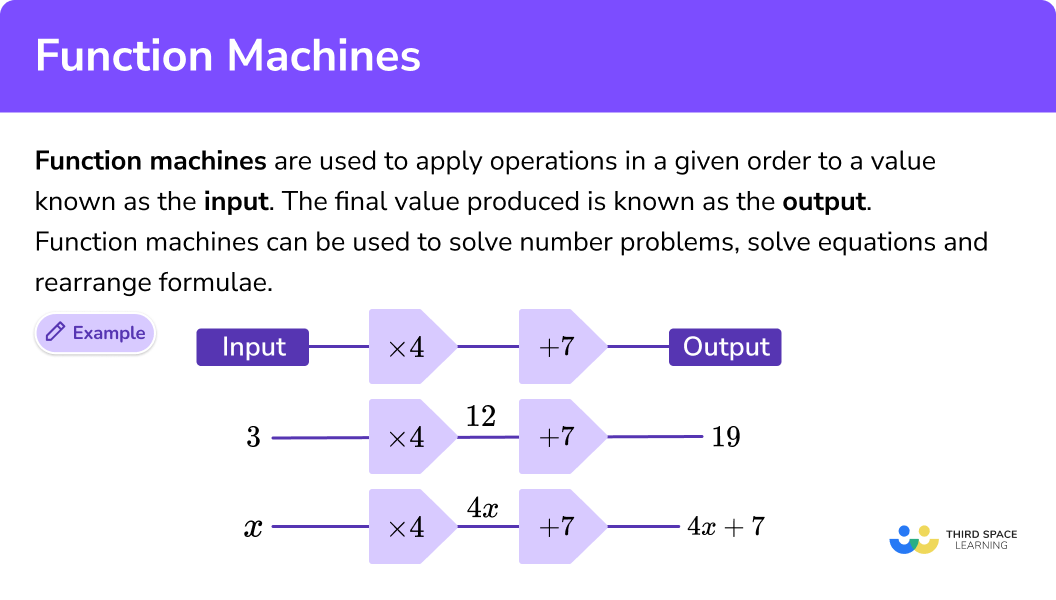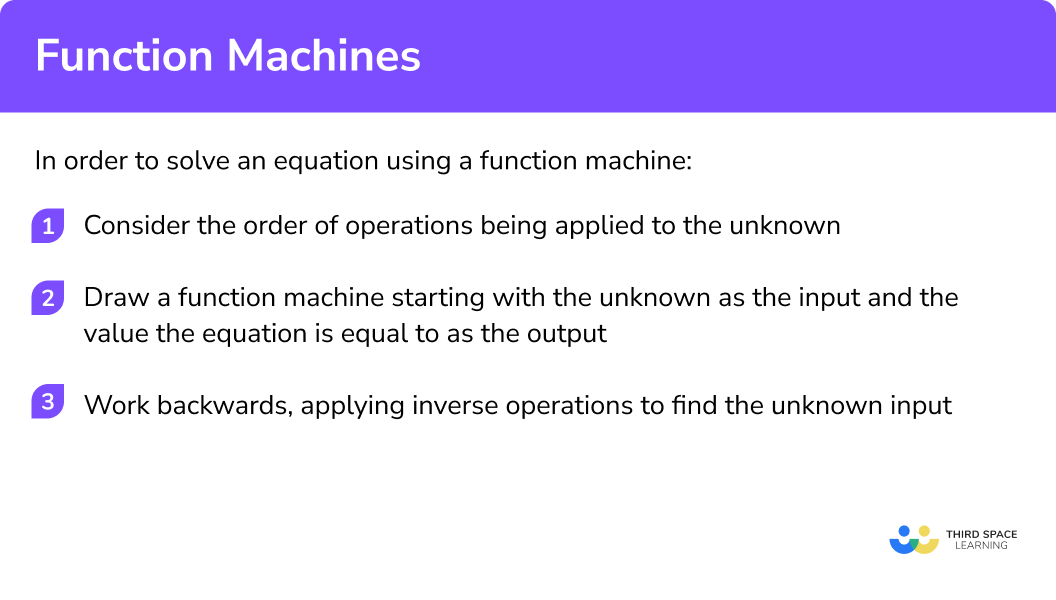GCSE Maths Algebra Functions

Function Machines

# Function Machines

Here we will learn about function machines, including finding outputs, finding inputs and using function machines to solve equations.

There are also function machine worksheets based on Edexcel, AQA and OCR exam questions, along with further guidance on where to go next if you’re still stuck.

## What are function machines?

Function machines are used to apply operations in a given order to a value known as the input. The final value produced is known as the output.

A function machine can be applied to numbers or be used for algebraic manipulation. They can be used to solve number problems, solve equations and rearrange formulae.

E.g.

To solve equations or rearrange formulae we need to use inverse operations and work backwards. We will see how to use function machines to solve equations on this page.

Not all equations can be solved using a function machine but they can be applied to a lot of situations where the unknown is on one side of the equation.

Function machines can be used to help produce tables of values for graphs such as quadratic or cubic graphs.

## Number machine

A number machine is an alternative name for function machines. A number machine is a way of writing the rules which link the inputs and the outputs.

### What are function machines?## How to solve equations using a function machine

In order to solve an equation using a function machine:

1. Consider the order of operations being applied to the unknown.
2. Draw a function machine starting with the unknown as the Input and the value the equation is equal to as the Output.
3. Work backwards, applying inverse operations to find the unknown Input.

### Explain how to solve equations using a function machine### Related lessons onfunctions in algebra

Function machines is part of our series of lessons to support revision on functions in algebra. You may find it helpful to start with the main functions in algebra lesson for a summary of what to expect, or use the step by step guides below for further detail on individual topics. Other lessons in this series include:

## Solving equations using function machines examples

### Example 1: solving a one-step-equation using a function machine

Solve x + 5 = 12

1. Consider the order of operations being applied to the unknown.

The only operation is + \;5 .

2Draw a function machine starting with the unknown as the Input and the value the equation is equal to as the Output.

3Work backwards, applying inverse operations to find the unknown Input.

x = 12 - 5

x = 7

### Example 2: solving a one-step-equation using a function machine

Solve 4x=20

Consider the order of operations being applied to the unknown.

Draw a function machine starting with the unknown as the Input and the value the equation is equal to as the Output.

Work backwards, applying inverse operations to find the unknown Input.

### Example 3: solving a two-step-equation using a function machine

Solve \frac{x}{2}-6=3

Consider the order of operations being applied to the unknown.

Draw a function machine starting with the unknown as the Input and the value the equation is equal to as the Output.

Work backwards, applying inverse operations to find the unknown Input.

### Example 4: solving a two-step-equation using a function machine

Solve 5\left( x+2 \right)=45

Consider the order of operations being applied to the unknown.

Draw a function machine starting with the unknown as the Input and the value the equation is equal to as the Output.

Work backwards, applying inverse operations to find the unknown Input.

### Example 5: solving a two-step-equation using a function machine

Solve \frac{3p-1}{4}=8

Consider the order of operations being applied to the unknown.

Draw a function machine starting with the unknown as the Input and the value the equation is equal to as the Output.

Work backwards, applying inverse operations to find the unknown Input.

### Example 6: solving a three-step-equation using a function machine

Solve \frac{2q+18}{3}=14

Consider the order of operations being applied to the unknown.

Draw a function machine starting with the unknown as the Input and the value the equation is equal to as the Output.

Work backwards, applying inverse operations to find the unknown Input.

### Common misconceptions

• Not working backwards when using inverse functions

When using two-step function machines or others with more operations to solve equations, a common error is to forget to work backwards. The inverse operations are used but in the wrong order.

• Not using the correct order of operations when drawing a function machine

A common error is to not follow the correct order of operations when creating a function machine for an equation.

E.g.
For the equation 2x-1=7 , the multiplication by two takes place before subtracting one.

### Practice function machines questions

1. Find the missing Output and missing Input for the function machine.a=17,\;b=35a=21,\;b=3a=17,\;b=3a=13,\;b=39Work forwards to find a and backwards, using inverse operations, to find b.

2. Find the missing Output and missing Input for the function machine.m=-1,\; n=84m=1,\; n=84m=12, \;n=28m=-12, \;n=28Work forwards to find m and backwards, using inverse operations, to find n.

3. Find the missing Output and missing Input for the function machine.p=8, \;q=9p=3, \;q=24p=24, \;q=3p=9, \;q=8Work forwards to find p and backwards, using inverse operations, to find q.

4. Select the correct the function machine for the equation:

5x=10x is the input, the operation is multiplying by 5 , the output is 10.

5. Select the correct the function machine for the equation:

2x-6=10x is the input, the operation is multiplying by 2 , the second operation is subtracting 6, the output is 10.

6. Select the correct function machine and solution to the equation.

2x-4=18Working backwards, you need to add four, and then divide by 2.

### Function machines GCSE questions

1. Here is a function machine(a) What is the output when the input is 6 ?

(b) What is the output when the input is 10 ?

(2 Marks)

(a) 40

(1)

(b) 68

(1)

2.  (a) Use the function machine to write a formula for y in terms of h. ?(b) Use inverse operations to write a formula for h in terms of y.

(6 Marks)

(a)

2h

(1)

2h+1

(1)

y=\frac{2h+1}{3}

(1)

(b)

3y

(1)

3y-1

(1)

h=\frac{3y-1}{2}

(1)

3.Fill in the missing numbers for the function machine.

(2 Marks)

First box 2

(1)

Second box 7

(1)

## Learning checklist

You have now learned how to:

• Use algebra to generalise the structure of arithmetic, including to formulate mathematical relationships
• Substitute values in expressions, rearrange and simplify expressions, and solve equations
• Recognise and use relationships between operations including inverse operations
• Where appropriate, interpret simple expressions as functions with inputs and outputs

## Still stuck?

Prepare your KS4 students for maths GCSEs success with Third Space Learning. Weekly online one to one GCSE maths revision lessons delivered by expert maths tutors.

Find out more about our GCSE maths tuition programme.